# Electronics and Communication Engineering - Power Electronics

### Exercise :: Power Electronics - Section 3

21.

In the below figure the rms voltage across each half of secondary is 200 V. The peak inverse voltage across each of thyristor is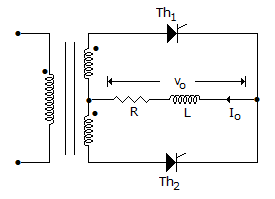A. 200 V B. 2(200) V C. 2(400) V D. 800 V

Explanation:

PIV = 2(2 x 200).

22.

Assertion (A): A GTO circuit is more compact than SCR circuit

Reason (R): A GTO can be turned off by negative gate pulse.

 A. Both A and R are correct and R is correct explanation of A B. Both A and R correct but R is not correct explanation of A C. A is correct but R is wrong D. A is wrong but R is correct

Explanation:

Since commutation circuit is not required, GTO circuit is compact.

23.

In a single phase full wave ac regulator, the firing angles in the two half cycles

 A. are always equal B. are sometimes equal C. are never equal D. may be equal or unequal

Explanation:

No answer description available for this question. Let us discuss.

24.

In a single phase full bridge converter (B - 2 connection), the input voltage is v = Vm sin ωt. The output dc voltage for resistive load

 A.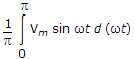B.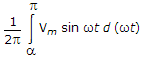C.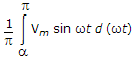D.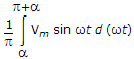Explanation:

SCRs conduct from a to p in each half cycle.

25.

As regards single phase dual converter

 A. the change in operation from one quadrant to the other is faster in non-circulating current mode B. the change in operation from one quadrant to the other is faster in circulating current mode C. the time response to change in operation from one quadrant to other is the same in both the modes D. the time response to change in operation from one quadrant to the other may be faster in any of the two modes